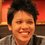# Prove the $(2n+1)$-tuple angle identity

It's common to know the triple angle formula, $\tan(3x) = \tan(x) \cdot \tan\left( x - \frac\pi3 \right) \cdot \tan\left( x + \frac\pi3 \right) .$ David Vreken pointed out in his unworldy solution here that quintuple angle formula below also holds! $\tan(5x) = \tan\left(\frac{2\pi}5 - x\right) \tan\left(\frac{\pi}5 - x\right) \tan(x) \tan\left(\frac{\pi}5 + x\right) \tan\left(\frac{2\pi}5 + x\right) .$ He even suggested that the following generalization must be true as well for any positive integer $n.$ Can anyone prove it? $\tan [(2n + 1)x] = (-1)^n \prod_{k=1}^{2n + 1} \tan\left(x + \frac{k - n - 1}{2n + 1}\pi \right)$Note by Pi Han Goh
2 months, 3 weeks ago

This discussion board is a place to discuss our Daily Challenges and the math and science related to those challenges. Explanations are more than just a solution — they should explain the steps and thinking strategies that you used to obtain the solution. Comments should further the discussion of math and science.

When posting on Brilliant:

• Use the emojis to react to an explanation, whether you're congratulating a job well done , or just really confused .
• Ask specific questions about the challenge or the steps in somebody's explanation. Well-posed questions can add a lot to the discussion, but posting "I don't understand!" doesn't help anyone.
• Try to contribute something new to the discussion, whether it is an extension, generalization or other idea related to the challenge.

MarkdownAppears as
*italics* or _italics_ italics
**bold** or __bold__ bold
- bulleted- list
• bulleted
• list
1. numbered2. list
1. numbered
2. list
Note: you must add a full line of space before and after lists for them to show up correctly
paragraph 1paragraph 2

paragraph 1

paragraph 2

[example link](https://brilliant.org)example link
> This is a quote
This is a quote
    # I indented these lines
# 4 spaces, and now they show
# up as a code block.

print "hello world"
# I indented these lines
# 4 spaces, and now they show
# up as a code block.

print "hello world"
MathAppears as
Remember to wrap math in $$ ... $$ or $ ... $ to ensure proper formatting.
2 \times 3 $2 \times 3$
2^{34} $2^{34}$
a_{i-1} $a_{i-1}$
\frac{2}{3} $\frac{2}{3}$
\sqrt{2} $\sqrt{2}$
\sum_{i=1}^3 $\sum_{i=1}^3$
\sin \theta $\sin \theta$
\boxed{123} $\boxed{123}$

Sort by:

We have the identities $\sin nx = 2^{n - 1} \prod_{k = 0}^{n - 1} \sin \left( x + \frac{k \pi}{n} \right)$ and $\cos nx = 2^{n - 1} \prod_{k = 1}^n \sin \left( x + \frac{(2k - 1) \pi}{2n} \right).$ If you divide these, you should be able to manipulate the quotient to get what you want.

References:

https://mathworld.wolfram.com/Multiple-AngleFormulas.html

- 2 months, 2 weeks ago

Here's a start to a proof:

Since $\tan x = \cfrac{e^{ix} - e^{-ix}}{i(e^{ix} + e^{-ix})} = \cfrac{e^{2ix} - 1}{i(e^{2ix} + 1)}$, that means that $\tan(x + a) = \cfrac{e^{2ix}e^{2ia} - 1}{i(e^{2ix}e^{2ia} + 1)}$. Therefore,

$\tan(\cfrac{\pi}{3} - x) \cdot \tan x \cdot \tan(\cfrac{\pi}{3} + x)$

$= -\tan(x - \cfrac{\pi}{3}) \cdot \tan x \cdot \tan(x + \cfrac{\pi}{3})$

$= -\cfrac{e^{2ix}e^{-\frac{2\pi}{3}i} - 1}{i(e^{2ix}e^{-\frac{2\pi}{3}i} + 1)} \cdot \cfrac{e^{2ix} - 1}{i(e^{2ix} + 1)} \cdot \cfrac{e^{2ix}e^{\frac{2\pi}{3}i} - 1}{i(e^{2ix}e^{\frac{2\pi}{3}i} + 1)}$

$= -\cfrac{e^{6ix} - e^{4ix}(e^{\frac{2\pi}{3}i} + e^{-\frac{2\pi}{3}i} + 1) + e^{2ix}(e^{\frac{2\pi}{3}i} + e^{-\frac{2\pi}{3}i} + 1) - 1}{i^3(e^{6ix} + e^{4ix}(e^{\frac{2\pi}{3}i} + e^{-\frac{2\pi}{3}i} + 1) + e^{2ix}(e^{\frac{2\pi}{3}i} + e^{-\frac{2\pi}{3}i} + 1) + 1)}$

$= \cfrac{e^{6ix} - e^{4ix}(0) + e^{2ix}(0) - 1}{i(e^{6ix} + e^{4ix}(0) + e^{2ix}(0) + 1)}$

$= \cfrac{e^{6ix} - 1}{i(e^{6ix} + 1)}$

$= \tan 3x$

Likewise,

$\tan(\cfrac{2\pi}{5} - x) \cdot \tan(\cfrac{\pi}{5} - x) \cdot \tan x \cdot \tan(\cfrac{\pi}{5} + x) \cdot \tan(\cfrac{2\pi}{5} + x)$

$= \tan(x - \cfrac{2\pi}{5}) \cdot \tan(x - \cfrac{\pi}{5}) \cdot \tan x \cdot \tan(x + \cfrac{\pi}{5}) \cdot \tan(x + \cfrac{2\pi}{5})$

$= \cfrac{e^{2ix}e^{-\frac{4\pi}{5}i} - 1}{i(e^{2ix}e^{-\frac{4\pi}{5}i} + 1)} \cdot \cfrac{e^{2ix}e^{-\frac{2\pi}{5}i} - 1}{i(e^{2ix}e^{-\frac{2\pi}{5}i} + 1)} \cdot \cfrac{e^{2ix} - 1}{i(e^{2ix} + 1)} \cdot \cfrac{e^{2ix}e^{\frac{2\pi}{5}i} - 1}{i(e^{2ix}e^{\frac{2\pi}{5}i} + 1)} \cdot \cfrac{e^{2ix}e^{\frac{4\pi}{5}i} - 1}{i(e^{2ix}e^{\frac{4\pi}{5}i} + 1)}$

$= \cfrac{e^{10ix} - e^{8ix}(e^{\frac{4\pi}{5}i} + e^{-\frac{4\pi}{5}i} + e^{\frac{2\pi}{5}i} + e^{-\frac{2\pi}{5}i} + 1) + e^{6ix}(e^{\frac{6\pi}{5}i} + e^{-\frac{6\pi}{5}i} + e^{\frac{4\pi}{5}i} + e^{-\frac{4\pi}{5}i} + 2e^{\frac{2\pi}{5}i} + 2e^{-\frac{2\pi}{5}i} + 2) - e^{4ix}(e^{\frac{6\pi}{5}i} + e^{-\frac{6\pi}{5}i} + e^{\frac{4\pi}{5}i} + e^{-\frac{4\pi}{5}i} + 2e^{\frac{2\pi}{5}i} + 2e^{-\frac{2\pi}{5}i} + 2) + e^{2ix}(e^{\frac{4\pi}{5}i} + e^{-\frac{4\pi}{5}i} + e^{\frac{2\pi}{5}i} + e^{-\frac{2\pi}{5}i} + 1) - 1}{i^5(e^{10ix} + e^{8ix}(e^{\frac{4\pi}{5}i} + e^{-\frac{4\pi}{5}i} + e^{\frac{2\pi}{5}i} + e^{-\frac{2\pi}{5}i} + 1) + e^{6ix}(e^{\frac{6\pi}{5}i} + e^{-\frac{6\pi}{5}i} + e^{\frac{4\pi}{5}i} + e^{-\frac{4\pi}{5}i} + 2e^{\frac{2\pi}{5}i} + 2e^{-\frac{2\pi}{5}i} + 2) + e^{4ix}(e^{\frac{6\pi}{5}i} + e^{-\frac{6\pi}{5}i} + e^{\frac{4\pi}{5}i} + e^{-\frac{4\pi}{5}i} + 2e^{\frac{2\pi}{5}i} + 2e^{-\frac{2\pi}{5}i} + 2) + e^{2ix}(e^{\frac{4\pi}{5}i} + e^{-\frac{4\pi}{5}i} + e^{\frac{2\pi}{5}i} + e^{-\frac{2\pi}{5}i} + 1) + 1)}$

$= \cfrac{e^{10ix} - e^{8ix}(e^{\frac{4\pi}{5}i} + e^{-\frac{4\pi}{5}i} + e^{\frac{2\pi}{5}i} + e^{-\frac{2\pi}{5}i} + 1) + 2e^{6ix}(e^{\frac{4\pi}{5}i} + e^{-\frac{4\pi}{5}i} + e^{\frac{2\pi}{5}i} + e^{-\frac{2\pi}{5}i} + 1) - 2e^{4ix}(e^{\frac{4\pi}{5}i} + e^{-\frac{4\pi}{5}i} + e^{\frac{2\pi}{5}i} + e^{-\frac{2\pi}{5}i} + 1) + e^{2ix}(e^{\frac{4\pi}{5}i} + e^{-\frac{4\pi}{5}i} + e^{\frac{2\pi}{5}i} + e^{-\frac{2\pi}{5}i} + 1) - 1}{i(e^{10ix} + e^{8ix}(e^{\frac{4\pi}{5}i} + e^{-\frac{4\pi}{5}i} + e^{\frac{2\pi}{5}i} + e^{-\frac{2\pi}{5}i} + 1) + 2e^{6ix}(e^{\frac{4\pi}{5}i} + e^{-\frac{4\pi}{5}i} + e^{\frac{2\pi}{5}i} + e^{-\frac{2\pi}{5}i} + 1) + 2e^{4ix}(e^{\frac{4\pi}{5}i} + e^{-\frac{4\pi}{5}i} + e^{\frac{2\pi}{5}i} + e^{-\frac{2\pi}{5}i} + 1) + e^{2ix}(e^{\frac{4\pi}{5}i} + e^{-\frac{4\pi}{5}i} + e^{\frac{2\pi}{5}i} + e^{-\frac{2\pi}{5}i} + 1) + 1)}$

$= \cfrac{e^{10ix} - e^{8ix}(0) + 2e^{6ix}(0) - 2e^{4ix}(0) + e^{2ix}(0) - 1}{i(e^{10ix} + e^{8ix}(0) + 2e^{6ix}(0) + 2e^{4ix}(0) + e^{2ix}(0) + 1)}$

$= \cfrac{e^{10ix} - 1}{i(e^{10ix} + 1)}$

$= \tan 5x$

Since $e^{\frac{-2n\pi}{2n + 1}i} + e^{\frac{-2(n - 1) \pi}{2n + 1}i} + ... + e^{\frac{-2 \pi}{2n + 1}i} + e^{\frac{0 \pi}{2n + 1}i} + e^{\frac{2\pi}{2n + 1}i} + ... + e^{\frac{2(n - 1)\pi}{n + 1}i} + e^{\frac{2n\pi}{2n + 1}i} = 0$ for any integer $n$, the above middle terms will always cancel out for the general case (more work needed here) and will prove the given generalization.

- 2 months, 2 weeks ago

I found the $\tan 5x$ equation here. There seems to be a start of a proof for it but I don't understand it. Perhaps someone can figure that out and generalize it.

- 2 months, 3 weeks ago

Summoning @Mark Hennings

- 2 months, 3 weeks ago